• 封装接口直接利用DataFrame绘制百分比柱状图1. 背景前言2. 官方网址示例2.1三级目录 手动反爬虫： 知识梳理不易，请尊重劳动成果，文章仅发布在CSDN网站上，在其他网站看到该博文均属于未经作者授权的恶意爬取信息 ...
封装接口直接利用DataFrame绘制百分比柱状图1. 背景前言2. 官方网址示例2.1 matplotlib_percentage_stacked_bar_plot2.2 percent-stacked-barplot2.3 Discrete distribution as horizontal bar chart3. 问题解决3.1 全部代码3.2 代码试错4. 实操检验4.1 实操测试4.2 更换标签4.3 部分标签输出
手动反爬虫：原博地址 https://editor.csdn.net/md/?articleId=106524459
 知识梳理不易，请尊重劳动成果，文章仅发布在CSDN网站上，在其他网站看到该博文均属于未经作者授权的恶意爬取信息

本文为付费内容，禁止转载
1. 背景前言
最近打比赛遇到的问题有点多，在绘制了堆叠柱状图之后，队长说不仅要看到具体的数量多少的堆叠图，还要看到具体占比的百分比柱状图，具体的样例可参考灵魂画图，因此也就产生了绘制百分比柱状图的需求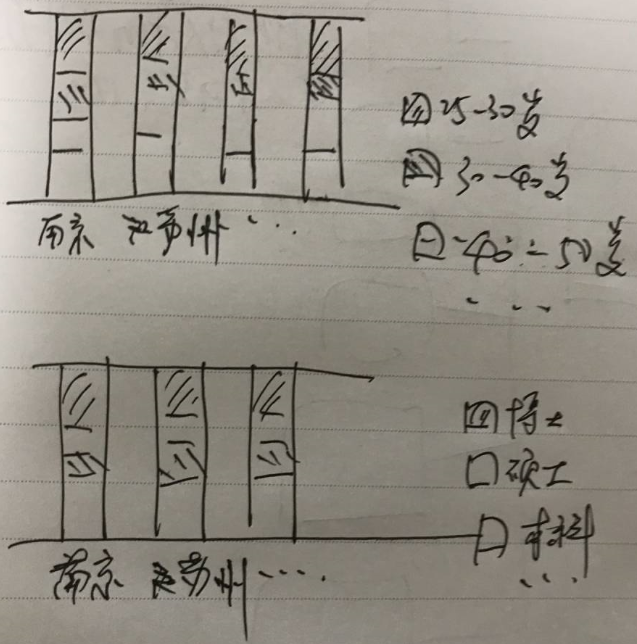2. 官方网址示例
2.1 matplotlib_percentage_stacked_bar_plot
示例网址1
里面的代码是真的长，完全属于一步步进行“堆砖块”然后才形成的百分比堆叠图，最终得出图结果如下：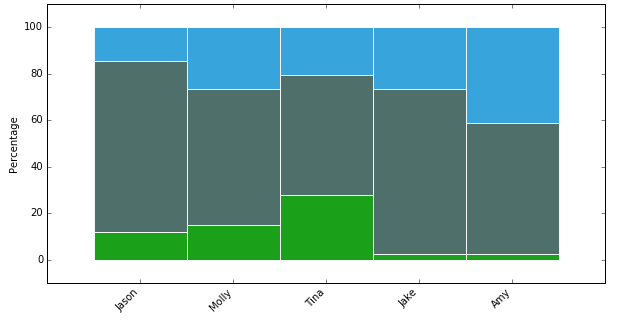2.2 percent-stacked-barplot
示例网址2
这个网址给出的代码比第一个网址给出的较为简洁，但是本质上都是属于一个个“砖块”的叠加，代码及生成的示例图形如下：
# libraries
import numpy as np
import matplotlib.pyplot as plt
from matplotlib import rc
import pandas as pd

# Data
r = [0,1,2,3,4]
raw_data = {'greenBars': [20, 1.5, 7, 10, 5], 'orangeBars': [5, 15, 5, 10, 15],'blueBars': [2, 15, 18, 5, 10]}
df = pd.DataFrame(raw_data)

# From raw value to percentage
totals = [i+j+k for i,j,k in zip(df['greenBars'], df['orangeBars'], df['blueBars'])]
greenBars = [i / j * 100 for i,j in zip(df['greenBars'], totals)]
orangeBars = [i / j * 100 for i,j in zip(df['orangeBars'], totals)]
blueBars = [i / j * 100 for i,j in zip(df['blueBars'], totals)]

# plot
barWidth = 0.85
names = ('A','B','C','D','E')
# Create green Bars
plt.bar(r, greenBars, color='#b5ffb9', edgecolor='white', width=barWidth)
# Create orange Bars
plt.bar(r, orangeBars, bottom=greenBars, color='#f9bc86', edgecolor='white', width=barWidth)
# Create blue Bars
plt.bar(r, blueBars, bottom=[i+j for i,j in zip(greenBars, orangeBars)], color='#a3acff', edgecolor='white', width=barWidth)

# Custom x axis
plt.xticks(r, names)
plt.xlabel("group")

# Show graphic
plt.show()

→ 输出的结果为：（这个结果相对好一点）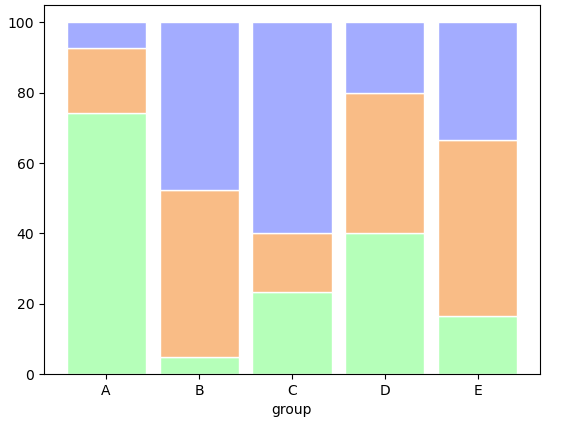2.3 Discrete distribution as horizontal bar chart
示例网址3
官方给出的示例图是下面这样的，看的第一眼是非常符合自己的要求的，而且还有不同的配色以及主题的标签设置及文本文字标注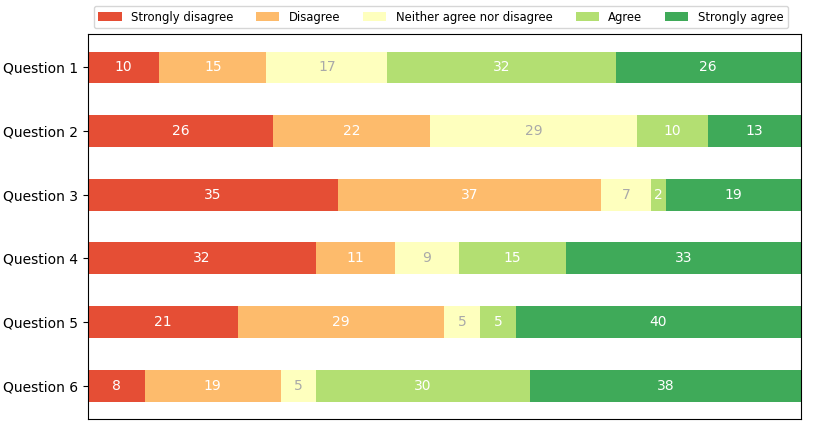接着就看一下给出的源码，如下，代码封装的挺不错的，还有可以直接调用的函数，按照官方示例的代码运行后的确可以实现上方的图形效果（内心狂喜~~ ）
import numpy as np
import matplotlib.pyplot as plt

category_names = ['Strongly disagree', 'Disagree',
'Neither agree nor disagree', 'Agree', 'Strongly agree']
results = {
'Question 1': [10, 15, 17, 32, 26],
'Question 2': [26, 22, 29, 10, 13],
'Question 3': [35, 37, 7, 2, 19],
'Question 4': [32, 11, 9, 15, 33],
'Question 5': [21, 29, 5, 5, 40],
'Question 6': [8, 19, 5, 30, 38]
}

def survey(results, category_names):
"""
Parameters
----------
results : dict
A mapping from question labels to a list of answers per category.
It is assumed all lists contain the same number of entries and that
it matches the length of *category_names*.
category_names : list of str
The category labels.
"""
labels = list(results.keys())
data = np.array(list(results.values()))
data_cum = data.cumsum(axis=1)
category_colors = plt.get_cmap('RdYlGn')(
np.linspace(0.15, 0.85, data.shape))

fig, ax = plt.subplots(figsize=(9.2, 5))
ax.invert_yaxis()
ax.xaxis.set_visible(False)
ax.set_xlim(0, np.sum(data, axis=1).max())

for i, (colname, color) in enumerate(zip(category_names, category_colors)):
widths = data[:, i]
starts = data_cum[:, i] - widths
ax.barh(labels, widths, left=starts, height=0.5,
label=colname, color=color)
xcenters = starts + widths / 2

r, g, b, _ = color
text_color = 'white' if r * g * b < 0.5 else 'darkgrey'
for y, (x, c) in enumerate(zip(xcenters, widths)):
ax.text(x, y, str(int(c)), ha='center', va='center',
color=text_color)
ax.legend(ncol=len(category_names), bbox_to_anchor=(0, 1),
loc='lower left', fontsize='small')

return fig, ax

survey(results, category_names)
plt.show()

这个官方给出的代码，看似可以解决问题，但是直接调用之后发现，并不是百分比柱状图的结果，比如这里将数据稍作修改，更改数据如下，然后再运行代码
results = {
'Question 1': [10, 15, 17, 32, 26],
'Question 2': [26, 22, 29, 10, 13],
'Question 3': [35, 37, 7, 2, 19],
'Question 4': [32, 11, 9, 15, 33],
'Question 5': [21, 29, 5, 5, 40],
'Question 6': [8, 190, 5, 30, 38] #在19后面加个0
}

→ 输出的结果为：（实际上这个图本身就不是百分比堆叠柱状图，根据官方的名称是离散分布的水平柱形图）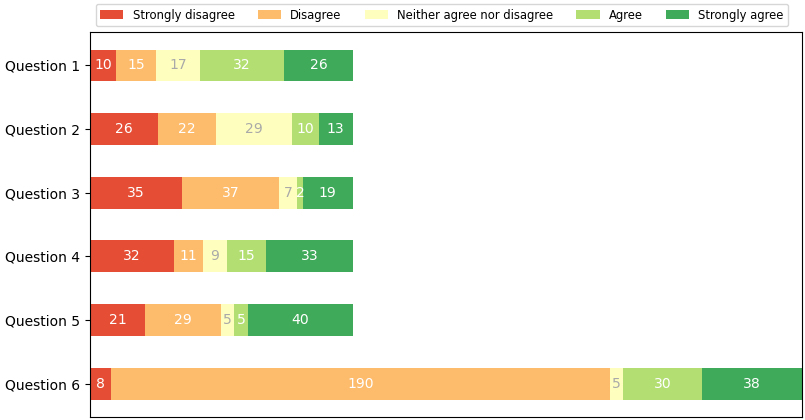然后百度搜索相关的python绘制的百分比堆叠柱状图基本上都是进行一个个“垒砌”的，看到百度搜索返回的第一个结果，竟然把这个官方的示例声称为自己封装的函数，标题还是绘制百分比柱状图，误导读者（本人也是被忽悠了，直到看到官网示例和实际调试）
3. 问题解决
既然没有现成的可以直接copy的代码，而且也没有像绘制堆叠图时候直接就拥有stacked的参数，所以就必须自己手动整理了。前面的第三个示例代码的框架可以直接拿过来用，就不用再进行编写了，只需要修改部分内容即可
3.1 全部代码
仿照之前的代码形式，进行代码改写，只需要传入DataFrame数据即可，如下
def percentage_bar(df):
labels = df.index.tolist() #提取分类显示标签
results = df.to_dict(orient = 'list') #将数值结果转化为字典
category_names = list(results.keys()) # 提取字典里面的类别（键-key）
data = np.array(list(results.values())) #提取字典里面的数值（值-value）

category_colors = plt.get_cmap('RdYlGn')(np.linspace(0.15, 0.85, data.shape))
#设置占比显示的颜色，可以自定义，修改括号里面的参数即可，如下
#category_colors = plt.get_cmap('hot')(np.linspace(0.15, 0.85, data.shape))

fig, ax = plt.subplots(figsize=(12, 9)) #创建画布，开始绘图
ax.invert_xaxis()#这个可以通过设置df中columns的顺序调整
ax.yaxis.set_visible(False) #设置y轴刻度不可见
ax.set_xticklabels(labels=labels, rotation=90) #显示x轴标签，并旋转90度
ax.set_ylim(0,1) #设置y轴的显示范围
starts = 0  #绘制基准
for i, (colname, color) in enumerate(zip(category_names, category_colors)):
heights = data[i,: ]/ data.sum(axis =0) #计算出每次遍历时候的百分比
ax.bar(labels, heights, bottom=starts, width=0.5,label=colname, color=color,edgecolor ='gray') # 绘制柱状图
xcenters = starts + heights/2  #进行文本标记位置的选定
starts += heights  #核心一步，就是基于基准上的百分比累加
#print(starts) 这个变量就是能否百分比显示的关键，可以打印输出看一下
percentage_text = data[i,: ]/ data.sum(axis =0) #文本标记的数据

r, g, b, _ = color  # 这里进行像素的分割
text_color = 'white' if r * g * b < 0.5 else 'k'  #根据颜色基调分配文本标记的颜色
for y, (x, c) in enumerate(zip(xcenters, percentage_text)):
ax.text(y, x, f'{round(c*100,2)}%', ha='center', va='center',
color=text_color, rotation = 90) #添加文本标记
ax.legend(ncol=len(category_names), bbox_to_anchor=(0, 1),
loc='lower left', fontsize='large') #设置图例
return fig, ax  #返回图像

比如把刚刚示例中的数据转化为DataFrame数据，如下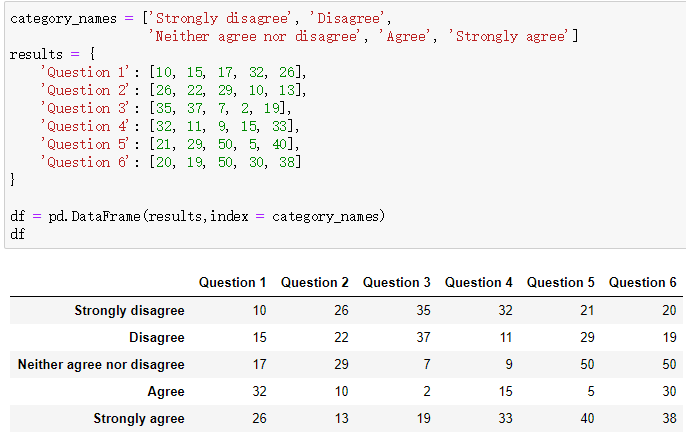然后将df作为参数直接传递到percentage_bar函数中，看看结果输出，内部虽然设置x轴标签旋转90度，但是在函数外边还是可以直接调整其方向，这里就是取消旋转了，而且在最后的文本标记中采用的是百分比进行进标注的，这样更能清晰的明确各方的占比情况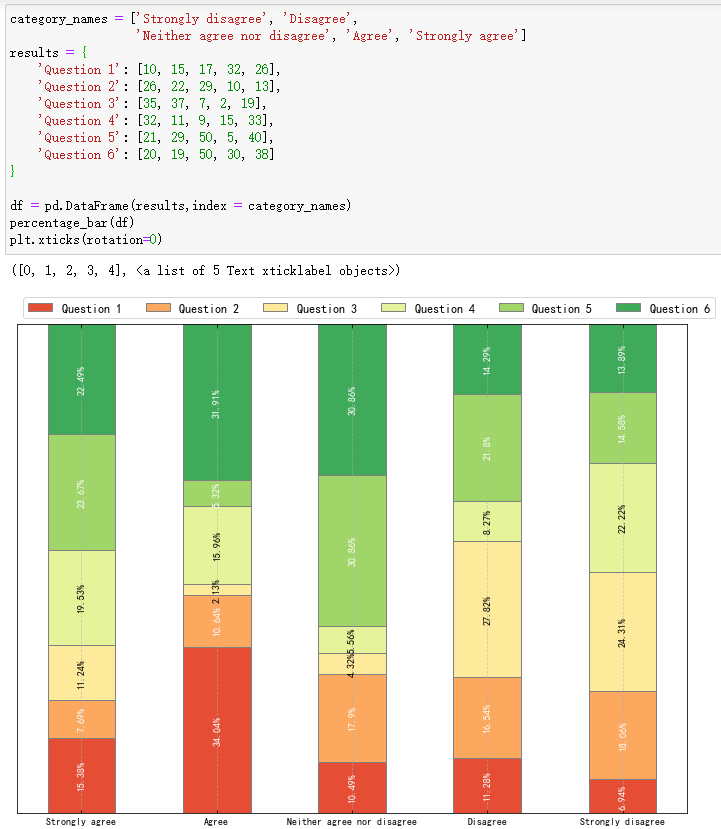3.2 代码试错
还是和最初一样，在最后一行数据的19后面添加一个0，变成190，这里顺带将y轴的刻度范围调整为（0,1.1），来查看是否符合百分比的要求，然后执行代码及输出结果如下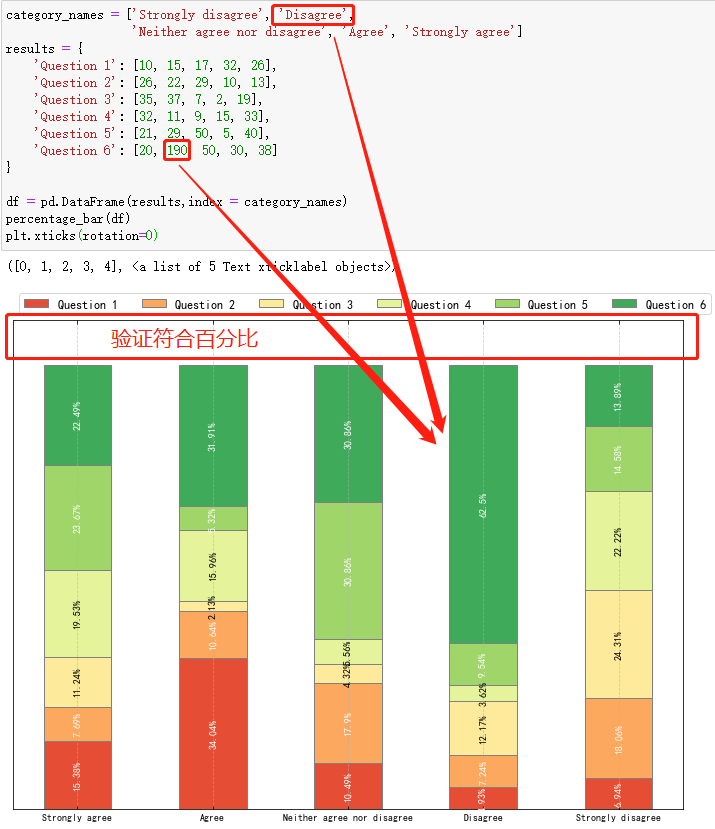4. 实操检验
上面已经对函数进行了试错，无误后直接将函数放置到自己自定义的第三方模块下，方便自己调用，关于这步的操作，可以参考博客将自定义常用的一些函数封装成可以直接调用的模块方法
4.1 实操测试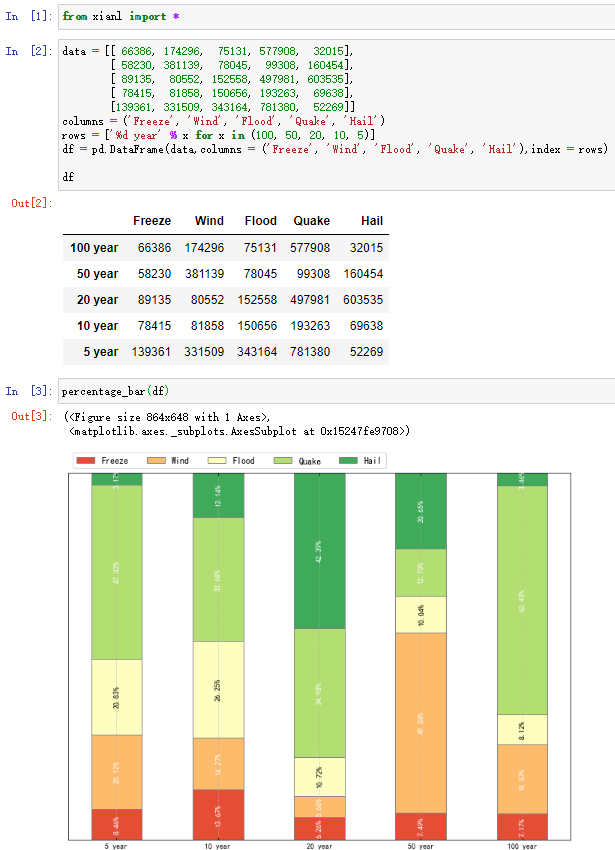4.2 更换标签
如果需要更换标签显示（df的标签与列标题互换），只需要将df转置即可，如下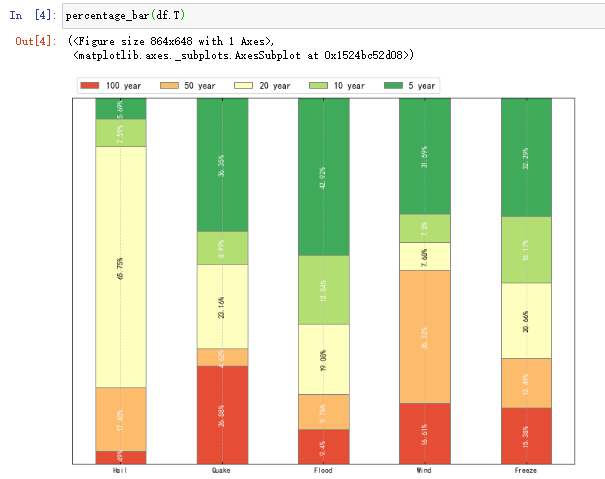4.3 部分标签输出
如果需要输出部分的标签，可以直接对df的columns进行切片即可（如果更换标签index转置之后也是同理），输出如下，比如这里只取两个标签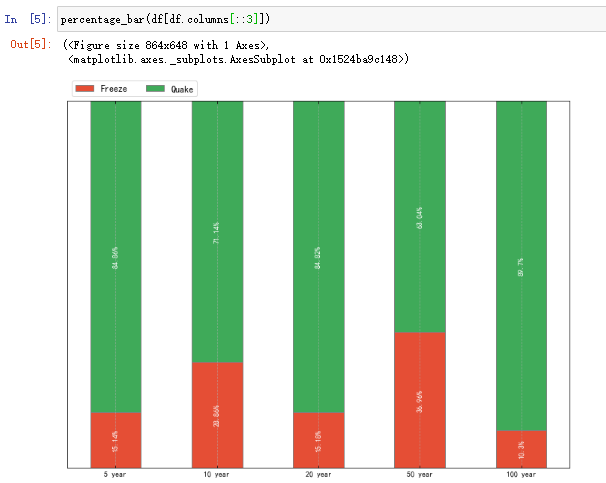最后，梳理不易，码字分享希望能够帮助大家。


展开全文python 数据可视化 数据分析 matplotlib
• 比如有两列数据 [img=... 假设4次，都是读了100次，，每次读的成功次数分别是，图上的四个值 ... 如何在下面的柱状图里，标识比例柱状？ 现在这个都是顶到顶部，全是100%了 谢谢
• ** series->setLabelsVisible(true);//设置数据是否可见 ** QT的官方柱状图，如下图 ...然后需要一根一根的柱子QBarSet，再把一根一根的柱子加到柱子的列当中QBarSeries，那么柱状图机画好了，可是没有x...
**
series->setLabelsVisible(true);//设置数据是否可见
**

QT的官方柱状图，如下图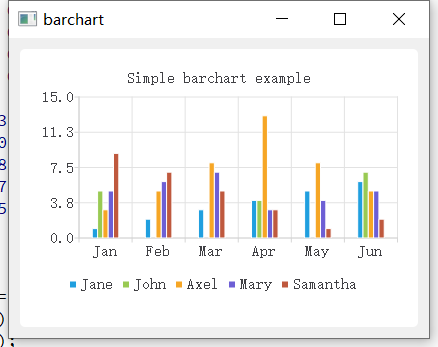QtCharts画图表必须要的两样东西QChartView，QChart相当于画布画笔，就不多说了。无论画什么图主要差别在于画笔里面的东西。
然后需要一根一根的柱子QBarSet，再把一根一根的柱子加到柱子的列当中QBarSeries，那么柱状图机画好了，可是没有x、y轴啊，哈哈哈，所有还需要为QChart和QBarSeries设置x、y轴。那么就完成了。具体实现如下：
int main(int argc, char *argv[])
{
QApplication a(argc, argv);

//!设置数据，相当于多少根柱子，每根的高度，
QBarSet *set0 = new QBarSet("Jane");
QBarSet *set1 = new QBarSet("John");
QBarSet *set2 = new QBarSet("Axel");
QBarSet *set3 = new QBarSet("Mary");
QBarSet *set4 = new QBarSet("Samantha");

*set0 << 1 << 2 << 3 << 4 << 5 << 6;
*set1 << 5 << 0 << 0 << 4 << 0 << 7;
*set2 << 3 << 5 << 8 << 13 << 8 << 5;
*set3 << 5 << 6 << 7 << 3 << 4 << 5;
*set4 << 9 << 7 << 5 << 3 << 1 << 2;
//!

//!把数据加载到数据列表中
QBarSeries *series = new QBarSeries();
series->append(set0);
series->append(set1);
series->append(set2);
series->append(set3);
series->append(set4);

//!

//!初始化画笔
QChart *chart = new QChart();
chart->setTitle("Simple barchart example");
chart->setAnimationOptions(QChart::SeriesAnimations);
//!

//!为QChart和QBarSeries设置坐标轴
QStringList categories;
categories << "Jan" << "Feb" << "Mar" << "Apr" << "May" << "Jun";
QBarCategoryAxis *axisX = new QBarCategoryAxis();//初始化X轴
axisX->append(categories);
series->attachAxis(axisX);

QValueAxis *axisY = new QValueAxis();//初始化Y轴
axisY->setRange(0,15);
series->attachAxis(axisY);
//!

//!设置图标的标题属性
chart->legend()->setVisible(true);
chart->legend()->setAlignment(Qt::AlignBottom);
//!

//!设置画布
QChartView *chartView = new QChartView(chart);
chartView->setRenderHint(QPainter::Antialiasing);
//!

//!
QMainWindow window;
window.setCentralWidget(chartView);
window.resize(420, 300);
window.show();
//!

return a.exec();
}

百分比柱状图，如下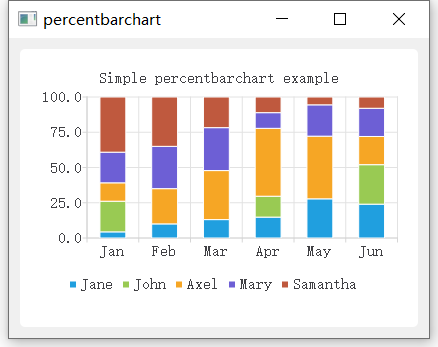做法跟上面的柱状图差不多，就是把数据序列QBarSeries 换成QPercentBarSeries而已，其它完全一致
横向百分比柱状图，如下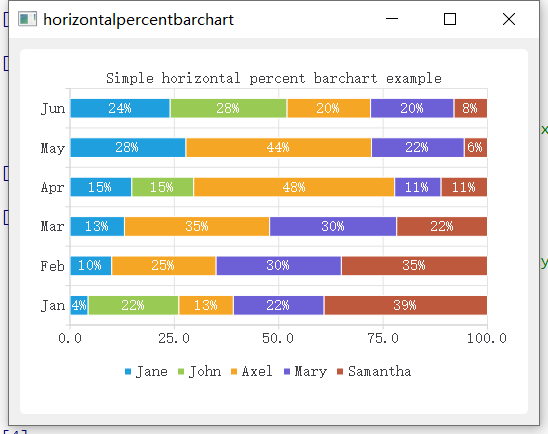做法跟上面的柱状图差不多，就是把数据序列QBarSeries 换成QHorizontalPercentBarSeries而已，其它完全一致


展开全文• 一款基于html5 canvas和jQuery绘制的3D水池百分比柱形图特效，点击柱状图可以动态改变水量高度值！jquery特效
• matplotlib之堆积柱状图百分比柱状图 在博主日常的绘图过程，有一种图也是什么常见的绘图工作，就是堆积的柱状图，其实很简单，就注意几个参数即可。 Here we go! 首先是引入数据库： import matplotlib.pyplot as...
matplotlib之堆积柱状图及百分比柱状图
在博主日常的绘图过程，有一种图也是什么常见的绘图工作，就是堆积的柱状图，其实很简单，就注意几个参数即可。 Here we go!
首先是引入数据库：
import matplotlib.pyplot as plt
import numpy as np
import pandas as pd

然后我创建了一个csv文件，这里随机填写了一些数据，详细如下：接下来用pandas读取数据，用pandas读取csv十分简单，几行代码搞定：
name = csv.Person
salary1 = np.array(csv.Salary1)
salary2 = np.array(csv.Salary2)
salary3 = np.array(csv.Salary3)

这里把pandas对象转成np的array是为了方便之后的array加减操作。
这里展示两种效果，因此用一下subplots函数创建了对比图
fig, axs = plt.subplots(1 , 2, figsize=(10,4))
axs.bar(index, salary1, width=0.4, label= 'salary1')
axs.bar(index, salary2, width=0.4, bottom=salary1, label= 'salary2')
axs.bar(index, salary3, width=0.4, bottom=salary2+salary1, label= 'salary3')
axs.set_ylim(0, 30000)
axs.set_xticklabels(name, rotation=90)

summ = salary1+salary2+salary3
percentage1 = salary1/summ
percentage2 = salary2/summ
percentage3 = salary3/summ

axs.bar(index, percentage1, width=0.4, label= salary1)
axs.bar(index, percentage2, width=0.4, bottom=percentage1, label= salary2)
axs.bar(index, percentage3, width=0.4, bottom=percentage1+percentage2, label= salary3)
axs.set_ylim(0,1)
axs.set_xticklabels(name, rotation=90)

plt.savefig('9.tiff', dpi=300)
plt.show()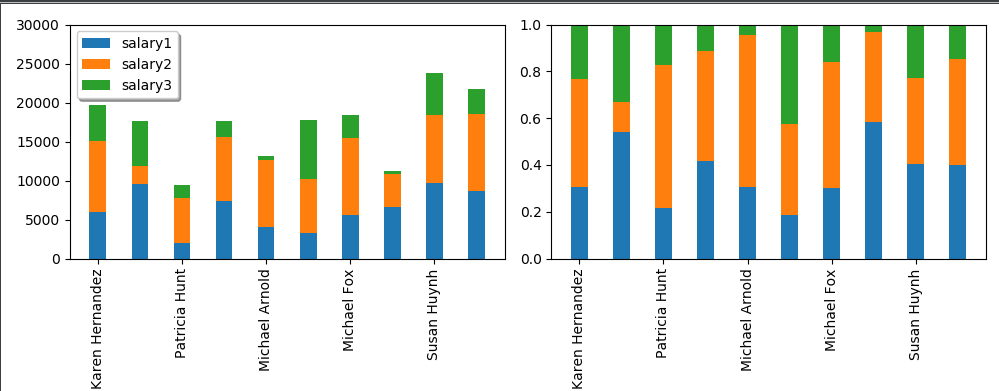这里左边显示了正常的堆积图，右边则显示了百分比堆积图，堆积的要点在于绘制时候的bottom关键字，这决定了柱状图的绘制起点，因此第二段的bottom是第一段的数值，而第三段则为第一、二段想加，
另外值得注意点 是，这里的subplots函数，返回对象有两个，前者fig相当于画布，而后者axs相当于在画布上绘制的元素，因此，我们这里是对绘制的元素操作，所以后续都是在ax上展开。

接下来还有一个需求，我们需要在柱状图上显示柱子对应的数值，比如右侧的图，需要加上红绿蓝颜色对应的百分比，那这里我们针对axs继续绘制。
这里先自定义一个函数:
def add_text(x, y, data):
for x0, y0, data0 in zip(x, y, data):
axs.text(x0, y0, round(data0, 1), rotation=90)

然后给出文本的坐标值以及数值即可：
注意这里绿色那段，由于柱子实在太短，如果要添加一下文本，需要手动微调位置，我这里就没继续添加了，
绘制图像如下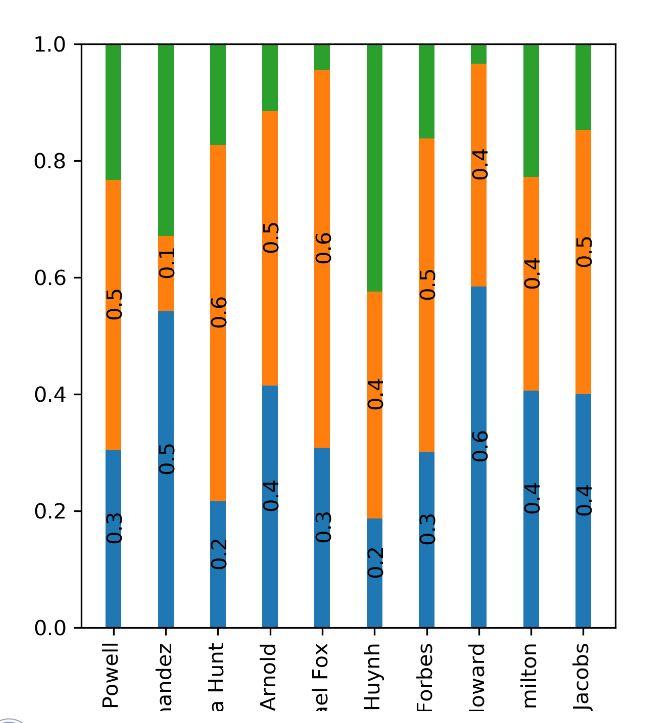完整代码如下：
import matplotlib.pyplot as plt
import numpy as np
import pandas as pd

for x0, y0, data0 in zip(x, y, data):
axs.text(x0, y0, round(data0, 1), rotation=90)

name = csv.Person
salary1 = np.array(csv.Salary1)
salary2 = np.array(csv.Salary2)
salary3 = np.array(csv.Salary3)
index = np.arange(len(name))
width = 0.4

fig, axs = plt.subplots(1, 2, figsize=(10, 5))
axs.bar(index, salary1, width=width, label='salary1')
axs.bar(index, salary2, width=width, bottom=salary1, label='salary2')
axs.bar(index, salary3, width=width, bottom=salary2 + salary1, label='salary3')
axs.set_ylim(0, 30000)
axs.set_xticks(index)
axs.set_xticklabels(name, rotation=90)

sum = salary1 + salary2 + salary3
percentage1 = salary1 / sum
percentage2 = salary2 / sum
percentage3 = salary3 / sum

axs.bar(index, percentage1, width=width, label=salary1)
axs.bar(index, percentage2, width=width, bottom=percentage1, label=salary2)
axs.bar(index, percentage3, width=width,
bottom=percentage1 + percentage2, label=salary3)
axs.set_ylim(0, 1)
axs.set_xticks(index)
axs.set_xticklabels(name, rotation=90)

y1 = percentage1/2
y2 = percentage1 + percentage2/2
y3 = sum - percentage3/2

plt.savefig('9.tiff', dpi=300)
plt.show()

更进一步的，写个自定义的函数，用来传输对象进去，直接调用函数试试：
以下为全代码：
import numpy as np
import matplotlib.pyplot as plt

category_names = ['Strongly disagree', 'Disagree',
'Neither agree nor disagree', 'Agree', 'Strongly agree']
results = {
'Question 1': [10, 15, 17, 32, 26],
'Question 2': [26, 22, 29, 10, 13],
'Question 3': [35, 37, 7, 2, 19],
'Question 4': [32, 11, 9, 15, 33],
'Question 5': [21, 29, 5, 5, 40],
'Question 6': [8, 19, 5, 30, 38]
}

def survey(results, category_names):
labels = list(results.keys())
# 获取标签
data = np.array(list(results.values()))
# 获取具体数值
data_cum = data.cumsum(axis=1)
# 逐项加和
category_colors = plt.get_cmap('RdYlGn')(np.linspace(0.15, 0.85, data.shape))

"""
在cmmap中取出五组颜色
category_colors:
[[0.89888504 0.30549789 0.20676663 1.        ]
[0.99315648 0.73233372 0.42237601 1.        ]
[0.99707805 0.9987697  0.74502115 1.        ]
[0.70196078 0.87297193 0.44867359 1.        ]
[0.24805844 0.66720492 0.3502499  1.        ]]

"""

print(category_colors)
# 常见颜色序列， 在cmap中取色

fig, ax = plt.subplots(figsize=(5, 9))
# 绘图
# ax.invert_xaxis()
# 使其更符合视觉习惯，index本身从下到上
ax.yaxis.set_visible(False)
ax.set_xticklabels(labels=labels, rotation=90)
# 不需要可见
ax.set_ylim(0, np.sum(data, axis=1).max())

for i, (colname, color) in enumerate(zip(category_names, category_colors)):
heights = data[:, i]
# 取第一列数值
starts = data_cum[:, i] - heights
# 取每段的起始点
ax.bar(labels, heights, bottom=starts, width=0.5,
label=colname, color=color)
xcenters = starts + heights / 2
r, g, b, _ = color
text_color = 'white' if r * g * b < 0.5 else 'darkgrey'
for y, (x, c) in enumerate(zip(xcenters, heights)):
ax.text(y, x, str(int(c)), ha='center', va='center',
color=text_color, rotation = 90)
ax.legend()
return fig, ax

survey(results, category_names)
plt.show()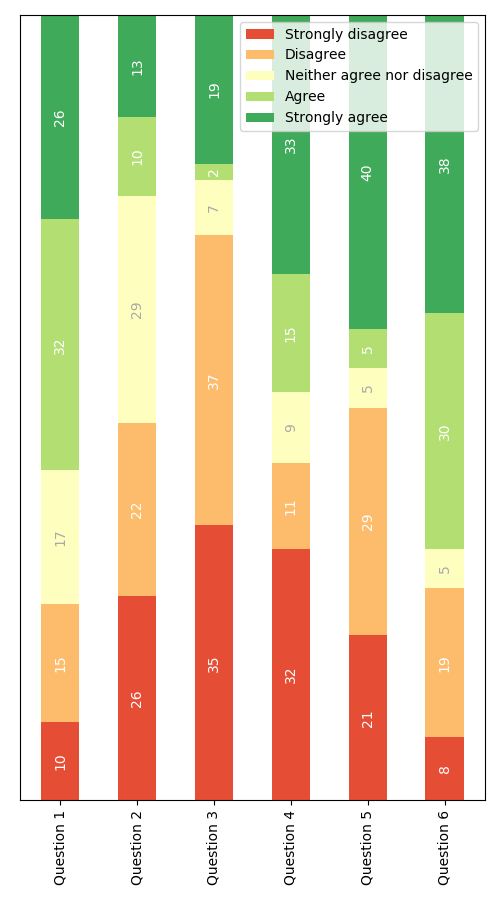完工！


展开全文• ## ECharts之横向百分比柱状图

千次阅读 多人点赞 2019-10-15 09:44:06
效果 背景图片 下载ECharts npm install echarts --save 引入并注册全局ECharts     在 main.js 文件里引入并注册 ( 这里是 Vue3.0 的模板 ) import Vue from 'vue' import App from './App.vue'...
效果图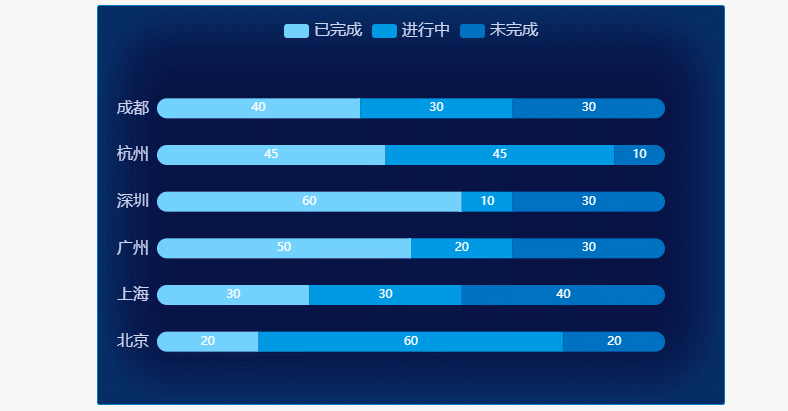背景图片下载ECharts
npm install echarts --save

引入并注册全局ECharts
在 main.js 文件里引入并注册 ( 这里是 Vue3.0 的模板 )
import Vue from 'vue'
import App from './App.vue'
import router from './router'
import store from './store'

import echarts from 'echarts'
Vue.prototype.$echarts = echarts Vue.config.productionTip = false new Vue({ router, store, render: h => h(App) }).$mount('#app')

在组件中使用ECharts
<template>
<div class='wrapper'>
<div class='chart' id='chart'></div>
</div>
</template>

<script>
export default {
data () {
return {}
},
mounted () {
this.drawChart()
},
methods: {
drawChart () {
// 基于准备好的dom，初始化echarts实例
let chart = this.\$echarts.init(document.getElementById('chart'))
// 监听屏幕变化自动缩放图表
window.addEventListener('resize', function () { chart.resize() })
// 绘制图表
chart.setOption({
color: ['#74d1fd', '#009ae4', '#0071c1'],
// 设置图表的位置
grid: {
x: 60, // 左间距
y: 80, // 上间距
x2: 60, // 右间距
y2: 40, // 下间距
containLabel: true // grid 区域是否包含坐标轴的刻度标签, 常用于『防止标签溢出』的场景
},
// 提示框组件
tooltip: {
trigger: 'axis', // 触发类型, axis: 坐标轴触发
axisPointer: {
// 指示器类型  'line' 直线指示器 'shadow' 阴影指示器 'none' 无指示器
// 'cross' 十字准星指示器 其实是种简写，表示启用两个正交的轴的 axisPointer
type: 'none'
},
textStyle: {
color: '#cdd3ee' // 文字颜色
},
// 提示框浮层内容格式器，支持字符串模板和回调函数两种形式 折线（区域）图、柱状（条形）图、K线图
// {a}（系列名称），{b}（类目值），{c}（数值）, {d}（无）
formatter: '{b}<br />{a0}: {c0}%<br />{a1}: {c1}%<br />{a2}: {c2}%'
},
// 图例组件
legend: {
textStyle: { // 文本样式
fontSize: 16,
color: '#cdd3ee'
},
top: 13, // 定位
data: [ '已完成', '进行中', '未完成' ] // 图例的数据数组
},
// X轴
xAxis: {
type: 'value', // 坐标轴类型,   'value' 数值轴，适用于连续数据
// 坐标轴刻度
axisTick: {
show: false // 是否显示坐标轴刻度 默认显示
},
// 坐标轴轴线
axisLine: { // 是否显示坐标轴轴线 默认显示
show: false // 是否显示坐标轴轴线 默认显示
},
// 坐标轴在图表区域中的分隔线
splitLine: {
show: false // 是否显示分隔线。默认数值轴显示
},
// 坐标轴刻度标签
axisLabel: {
show: false // 是否显示刻度标签 默认显示
}
},
yAxis: [
// 左侧Y轴
{
// 坐标轴类型,  'category' 类目轴，适用于离散的类目数据
// 为该类型时必须通过 data 设置类目数据
type: 'category',
// 坐标轴刻度
axisTick: {
show: false // 是否显示坐标轴刻度 默认显示
},
// 坐标轴轴线
axisLine: { // 是否显示坐标轴轴线 默认显示
show: false, // 是否显示坐标轴轴线 默认显示
lineStyle: { // 坐标轴线线的颜色
color: '#cdd3ee'
}
},
// 坐标轴在图表区域中的分隔线
splitLine: {
show: false // 是否显示分隔线。默认数值轴显示
},
// 坐标轴刻度标签
axisLabel: {
show: true, // 是否显示刻度标签 默认显示
fontSize: 16, // 文字的字体大小
color: '#cdd3ee', // 刻度标签文字的颜色
// 使用字符串模板，模板变量为刻度默认标签 {value}
formatter: '{value}'
},
// 类目数据，在类目轴（type: 'category'）中有效
data: ['北京', '上海', '广州', '深圳', '杭州', '成都']
}
],
// 系列列表
series: [
{
type: 'bar', // 系列类型
name: '已完成', // 系列名称, 用于tooltip的显示, legend 的图例筛选
// 数据堆叠，同个类目轴上系列配置相同的stack值后，后一个系列的值会在前一个系列的值上相加
stack: '总量',
barMaxWidth: 20, // 柱条的最大宽度，不设时自适应
// 图形上的文本标签
label: {
show: true,
// 标签的位置 left right bottom top inside  // 绝对的像素值 position: [10, 10]
// 相对的百分比 position: ['50%', '50%']
position: 'inside'
},
// 图形样式
itemStyle: {
barBorderRadius: [10, 0, 0, 10] // 圆角半径, 单位px, 支持传入数组分别指定 4 个圆角半径
},
data: [20, 30, 50, 60, 45, 40] // 系列中的数据内容数组
},
{
type: 'bar', // 系列类型
name: '进行中', // 系列名称, 用于tooltip的显示, legend 的图例筛选
// 数据堆叠，同个类目轴上系列配置相同的stack值后，后一个系列的值会在前一个系列的值上相加
stack: '总量',
barMaxWidth: 20, // 柱条的最大宽度，不设时自适应
// 图形上的文本标签
label: {
show: true,
// 标签的位置 left right bottom top inside  // 绝对的像素值 position: [10, 10]
// 相对的百分比 position: ['50%', '50%']
position: 'inside'
},
data: [60, 30, 20, 10, 45, 30] // 系列中的数据内容数组
},
{
type: 'bar', // 系列类型
name: '未完成', // 系列名称, 用于tooltip的显示, legend 的图例筛选
// 数据堆叠，同个类目轴上系列配置相同的stack值后，后一个系列的值会在前一个系列的值上相加
stack: '总量',
barMaxWidth: 20, // 柱条的最大宽度，不设时自适应
// 图形上的文本标签
label: {
show: true,
// 标签的位置 left right bottom top inside  // 绝对的像素值 position: [10, 10]
// 相对的百分比 position: ['50%', '50%']
position: 'inside'
},
// 图形样式
itemStyle: {
barBorderRadius: [0, 10, 10, 0] // 圆角半径, 单位px, 支持传入数组分别指定 4 个圆角半径
},
data: [20, 40, 30, 30, 10, 30] // 系列中的数据内容数组
}
]
})
}
}
}
</script>

<style scoped>
.wrapper {
width: 100%;
}
.wrapper .chart {
width: 60%;
height: 400px;
margin: 100px auto 0;
background: url(../../public/static/bg.png) no-repeat;
background-size: 100% 100%;
}
</style>




展开全文echarts vue.js javascript es6
• 这样的图一般难在折线图的数据很小，是百分比趋势图，所以经常相对前面主数据太小了，在图里看不到，所以需要用次坐标轴来将其显示出来，下面就介绍这种柱状图添加折线图（百分比趋势图）组合的做法。Excel百分比...
• 百分比堆积柱形图和三维百分比堆积柱形图 这些类型的柱形图比较各个类别的每一数值所占总数值的百分比大小。百分比堆积柱形图以二维垂直百分比堆积矩形显示数值...本节将以图3-7-1为例讲解百分比堆积柱形图制作过程。Excel 数据可视化
• 使用matplotlib创建百分比堆积柱状图的思路与堆积柱状图类似，只不过bottom参数累计的不是数值而是百分比，因此，需要事先计算每组柱子的数值总和，进而求百分比。 未使用numpy版本 适用于少量数据，数据结构需要...matplotlib pyplot numpy
• 这样的图一般难在折线图的数据很小，是百分比趋势图，所以经常相对前面主数据太小了，在图里看不到，所以需要用次坐标轴来将其显示出来，下面就介绍这种柱状图添加折线图（百分比趋势图）组合的做法。 Excel百分比...
• 用ggplot2画百分比柱状图 ## 添加字体 library(showtext) ## 为了使ggplot中有中文字体，可根据自己电脑里字体的位置自行添加。 font_add("kt", "/Users/cpf/Library/Fonts/楷体_GB2312.ttf") showtext_auto() # ...
• <html lang="en"> <head> <meta charset="UTF-8" /> , initial-scale=1.0" /> <meta http-equiv="X-UA-Compatible" content="ie=edge" /> 百分比-堆叠柱状图title> head> <body> <div id="container">div> <script> ...前端
• 目录1 问题2 从柱状图绘制完整统计图注意事项3 Python3代码4 参考文献1 问题同样是统计图，有的美观的表达了想法，有的却让人看不明白。那么，我们在绘制完整统计图时要注意哪些事项呢？下面通过柱状图来说明。2 ...
• 1.简介 用Origin8绘制百分比堆积柱形图。图中的数据Type1和Type2分别表示两个类型的数据所占的比例。 2.画图步骤（1）新建worksheet表，添加相关数据。然后选中全部数据（一个X多个Y），plot-Columns/Bars-Stack ...
• 文章目录pandas中的绘图函数内容概要线形图柱状图直方图随机百分比密度图散布图机器学习使用的比较多的一种图形散布图可以描述数据的分布...
• 随便画一个百分比堆积柱状图 活不多说直接上代码： library('ggplot2') #加载所需的包 data.diet <- data.frame(fre = c(7,71,33,45), diet = c('Omnivore', 'PlantSeed', 'Omnivore', 'PlantSeed'), beh = c('L'...r语言 ggplot2
• 上面展示了两种方法来绘制百分比图， 从效果来看，ggstatsplot的ggbarstats()函数更好，不仅直接展示了每个clarity里面各个color的占比，还在图形的左侧展示了每个clarity的样本量。比geom_bar()包含的信息量更多，...R语言 ggplot2
• 下面我就介绍一下如何制作柱形图图表完美呈现百分比，提升您报表的颜值。 具体步骤： 1、给表格添加一列辅助列，如下图： 2、插入柱形图，即选取A列、D列、E列，点击菜单栏的【插入】—【二维柱形图】； 3、插入的...
• 文章目录pandas中的绘图函数内容概要线形图柱状图直方图随机百分比密度图散布图机器学习使用的比较多的一种图形散布图可以描述数据的分布状态和数据的增长趋势以及x和y轴的关系汽车排放量越小，行驶的距离就越远 ...数据挖掘 python 机器学习 人工智能 数据分析
• 主要有两种方法： 1.ggplot，包裹器里添加fill 2.使用ggbarstats，会显示占比 library(ggplot2) #加载ggplot2包 library(dplyr) #加载dplyr包 library(ggstatsplot) #加载ggstatsplot包 diamonds2 <...
• 文章目录柱状图一个简单柱状图改变其颜色设置标签堆叠柱状图横向条形图并列柱状图饼状图简单饼状图一块饼图到中心距离设置颜色显示百分比 柱状图 一个简单柱状图 import matplotlib.pyplot as plt num_list=[1,5,6.5...
• QT绘制水平百分比条形 项目简介 项目技术 项目展示 主要源码片段解析 获取完整项目源码传送门 项目简介 创建简单的水平百分比条形。 水平百分比条形将集合中的数据显示为每个类别中所有集合的百分比。 创建...
• 如下代码： #导入扩展包 import matplotlib.pyplot as plt ...#设置柱状图的宽度 width = 0.4 #绘图 plt.figure(figsize=(8,4)) plt.bar(x=x,height=y1,width=width,label='Data1') plt.bar(x=x+Python...